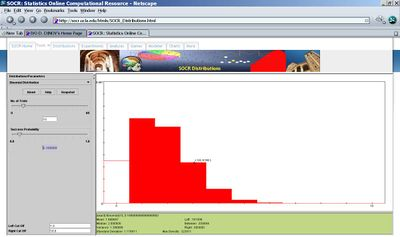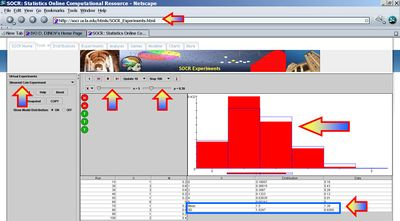# AP Statistics Curriculum 2007 Distrib Binomial

## General Advance-Placement (AP) Statistics Curriculum - Bernoulli and Binomial Random Variables and Experiments

### Bernoulli process

A Bernoulli trial is an experiment whose dichotomous outcomes are random (e.g., "success" vs. "failure", "head" vs. "tail", +/-, "yes" vs. "no", etc.) Most common notations of the outcomes of a Bernoulli process are success and failure, even though these outcome labels should not be construed literally.

• Examples of Bernoulli Trials
• A Coin Toss. We can obverse H="heads", conventionally denoted success, or T="tails" denoted as failure. A fair coin has the probability of success 0.5 by definition.
• Rolling a Die: The outcome space is binarized to "success"={6} and "failure" = {1, 2, 3, 4, 5}.
• Polls: Choosing a voter at random to ascertain whether that voter will vote "yes" in an upcoming referendum.
• The Bernoulli random variable (RV): Mathematically, a Bernoulli trial is modeled by a random variable $$X(outcome) = \begin{cases}0,& s = \texttt{failure},\\ 1,& s = \texttt{success}.\end{cases}$$

If p=P(success), then:

expected value of X, E[X]=p
standard deviation of X, SD[X] is $$\sqrt{p(1-p)}$$.
• A Bernoulli Process consists of repeatedly performing independent but identical Bernoulli trials.

### Binomial Random Variables

Suppose we conduct an experiment observing an n-trial (fixed) Bernoulli process. If we are interested in the RV X={Number of successes in the n trials}, then X is called a Binomial RV and its distribution is called Binomial Distribution.

#### Examples

• Roll a standard die ten times. Let X be the number of times {6} turned up. The distribution of the random variable X is a binomial distribution with n = 10 (number of trials) and p = 1/6 (probability of "success={6}). The distribution of X may be explicitly written as (P(X=x) are rounded of, you can compute these exactly by going to SOCR Distributions and selecting Binomial):
 x 0 1 2 3 4 5 6 7 8 9 10 P(X=x) 0.162 0.323 0.291 0.155 0.0543 0.013 0.0022 0.00025 1.9e-05 8.269e-07 1.654e-08• Suppose 10% of the human population carries the green-eye allele. If we choose 1,000 people randomly and let the RV X be the number of green-eyed people in the sample. Then the distribution of X is binomial distribution with n = 1,000 and p = 0.1 (denoted as $$X \sim B(1,000, 0.1)$$. In a sample of 1,000, how many of them are we expecting to have this allele?

### Binomial Modeling

#### Exact Binomial Model

The Binomial distribution (i.e., biased-coin tossing experiment) is an exact physical model for any experiment which can be characterized as a series of trials where:

• Each trial has only two outcomes: success or failure;
• P(success)=p is the same for every trial; and
• Trials are independent.

#### Approximate Binomial Model

Suppose we observe an experiment comprised of n identical trials involving a large population (of size N). Assume the population contains a sub-population of subjects that have a characteristic of interest and the sub-population proportion is p (0<p<1). Then the distribution of X={the number of outcomes in the sample with that characteristic of interest}, is approximately Binomial(n, p). This approximation is adequate if the ratio n/N < 0.05.

• Example: Polling the US population to see what proportion is/has-been married. Since we sample without replacement (can't ask the same individual twice), the second assumption of the (exact) Binomial model is (slightly) violated. Yet, the small ration of sample to population size yields that the Binomial model is approximately valid (i.e., the proportion of subjects that is/has-been married does not change significantly as we poll one subject, and therefore remove him/her from the complete pool of subjects we poll).

### Binomial Probabilities

If the random variable X follows the Binomial distribution with (fixed) parameters n (sample-size) and p (probability of success at one trial), we write X ~ B(n, p). The probability of getting exactly x successes is given by the Binomial probability (or mass) function$P(X=x)={n\choose x}p^x(1-p)^{n-x}$, for x = 0, 1, 2, ..., n, where $${n\choose x}=\frac{n!}{x!(n-x)!}$$ is the binomial coefficient.

This probability expression has an easy and intuitive interpretation. The probability of the x successes in the n trials is (px). Similarly, the probability of the n-x failures is (1 − p)n-x. However, the x successes can be arranged anywhere among the n trials, and there are $${n\choose x}=\frac{n!}{x!(n-x)!}$$ different ways of arranging the x successes in a sequence of n trials, see the Counting section.

See this Binomial distribution calculator.
See this Binomial coefficient calculator

### Binomial Expectation and Variance

If X is the random variable representing the r(random) number of heads in n coin toss trials, where the P(Head) = p, i.e., $$X\sim B(n, p)$$. Then we have the following expressions for the expected value, variance and the standard deviation of X :

### Examples

#### Binomial Coin Toss Example

Refer to the SOCR Binomial Coin Toss Experiment and use the SOCR Binomial Coin Toss Applet to perform an experiment of tossing a biased coin, P(Head) = 0.3, 5 times and comput the expectation of the number of Heads in such experiment. You should recognize that there are two distinct ways of computing the expected number of Heads:

• Theoretical calculation, using the Binomial Probabilities;$$E[X]=\sum_x{xP(X=x)} =$$

$$=0\times{5\choose 0}0.3^0(0.7)^{5}+1\times{5\choose 1}0.3^1(0.7)^{4}+2\times{5\choose 2}0.3^2(0.7)^{3}+3\times{5\choose 3}0.3^3(0.7)^{2}+4\times{5\choose 4}0.3^4(0.7)^{1}+5\times{5\choose 5}0.3^5(0.7)^{0} =$$ $$\cdots = (n\times p) = 5\times 0.3 = 1.5.$$

• Empirical calculation, using the outcomes 100 repeated coin tosses of 5 coins. The image below illustrates this approximate calculation of the expectation for the number of heads when $$X\sim B(5, 0.3)$$. Notice the slight difference between the theoretical expectation ($$n\times p = 5 \times 0.3 = 1.5$$) and its empirical approximation of 1.39!• Notes: Of course, the theoretical calculation is exact and the empirical calculation is only approximate. However, the power of the empirical approximation to the expected number of Heads becomes clearer when we increase the number of coins from 5 to 100, or more. In these cases, the exact calculations become very difficult, and for even larger number of coins become intractable.